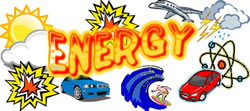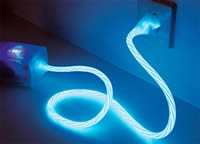# Difference between Energy and Power

##### Key difference: Energy is the capacity for doing work. It is the measure of how much fuel is contained within something, of how much work can it accomplish. It is the amount of power consumed in accomplishing work. Power, on the other hand, is the rate at which energy is used or consumed. It is the rate at which the work is accomplished. Essentially power is the energy used over time.Energy and power are two words we commonly use everyday. In common parlance, the terms have come be used interchangeably. However, that is not the case. The terms as actually used in physics denote something differently. They are interrelated however.

Energy is the capacity for doing work. It is the measure of how much fuel is contained within something, of how much work can it accomplish. It is the amount of power consumed in accomplishing work. Energy cannot be generated or used up. It can only be changed from one thing to another, i.e. the potential of accomplishing work is converted into actual work, or that energy is converted to heat.

Energy is required to do anything and everything. We use energy to lift our hand, we use energy to get up off the bed, we use energy in the form of electricity to run the light, we use energy to heat up the water, we use energy to heat or cool our homes, etc. Everything has energy that can be harnessed. In order to perform any work or carry out a change, first the required energy is needed. To do 100 joules of work, one needs to expend 100 joules of energy.

Joule (J) is a unit of energy. It can be used to denote how much energy there is in a substance. In addition to joule, some other units of energy include watt-hours (Wh), kilowatt-hours (kWh), British Thermal Units (BTU), the therm, calorie, kilocalorie, and many more.

Energy exists in many forms. It is mainly categorized as potential energy, kinetic energy or a mixture of both. These are further categorized as:

• Thermal energy, thermal energy in transit is called heat
• Chemical energy
• Electric energy
• Nuclear energy• Magnetic energy
• Elastic energy
• Sound energy
• Mechanical energy
• Luminous energy
• Mass (E=mc²)

Power, on the other hand, is the rate at which energy is used or consumed. It is the rate at which the work is accomplished. Essentially power is the energy used over time. (P = J/s). For example, if one does 100 joules of work in one second using 100 joules of energy, the power is 100 watts. Watt (W) is the SI unit of power, which is equal to one joule per second. Another unit for power is the kilowatt (kW), where one kW is equal to 1000 watts.

Image Courtesy: tiki.oneworld.net, organisationdevelopment.org